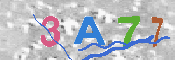# Project Euler – Problem 2 (Haskell vs C#)

19 Jun 2011

Problems with the .NET 3.5 implementation

During implementation of the same algorithm from Haskell to (Functional) C#, I found that I hit quite a large snag with .NET (3.5) number types. A fibonacci sequence very quickly accumulates into integers larger than an Unsigned Long can contain, in which case you would have to move to using .NET 4.0 / F# BigInt to get the benefits of using their operators. The below code works very well within the problem domain, however you will need to refactor this to use BigInt if you wish to move into larger Fibonnaci sequences.

Project Euler – Problem 2

Each new term in the Fibonacci sequence is generated by adding the previous two terms. By starting with 1 and 2, the first 10 terms will be:
1, 2, 3, 5, 8, 13, 21, 34, 55, 89, …
By considering the terms in the Fibonacci sequence whose values do not exceed four million, find the sum of the even-valued terms.

```module Main where main = do let fibs = map fst \$ iterate(\(a,b) -> (b,a+b)) (1,2) print \$ sum(takeWhile(< 4000000) (filter even fibs))```
Function Description
\ The backslash in this context is representing that the following code is a lambda (λ)
iterate Creates an infinite list where the first item is calculated by applying the function on the secod argument, the second item by applying the function on the previous result and so on.
\$ The ‘\$’ operator is for substituting parenthesis. Anything appearing after it will take precedence over anything that comes before.
fst Returns the first item in a tuple eg. fst (1,2) returns 1
map Returns a list constructed by applying a function (the first argument) to all items in a list passed as the second argument
filter returns a list constructed from members of a list (the second argument) fulfilling a condition given by the first argument
even returns True if the integral is even, False otherwise.
takeWhile creates a list from another one, it inspects the original list and takes from it its elements to the moment when the condition fails, then it stops processing
sum computes a sum of all elements in the list

Armed with the knowledge of what each of the above functions do, we can now translate the above code. Like most functional programs we read the code right to left meaning that the code on the right is passed to the code to the left of the chain.

given a list of even fibonnaci numbers
whose number doesn’t exceed 4 million
return the sum

Which is almost identical to the c# implementation using a YCombinator.

C# Implementation using YCombinator

```public void Main() { var sum = Fibbonacci(1, 2, Int32.MaxValue) .TakeWhile(x => x < 4000000) .Where(x => x % 2 == 0) .Sum();   Console.WriteLine(sum); Console.ReadLine(); }   private delegate Func<TA, TB, TC, TR> Recursive<TA, TB, TC, TR>(Recursive<TA, TB, TC, TR> r);   // Y Combinator private static Func<TA, TB, TC, TR> Y<TA,TB,TC,TR>(Func<Func<TA,TB,TC,TR>, Func<TA,TB,TC,TR>> f) { Recursive<TA,TB,TC,TR> rec = r => (a, b, c) => f(r(r))(a, b, c); return rec(rec); }   private readonly Func<long, long, long, IEnumerable<long>> Fibbonacci = Y<long, long, long, IEnumerable<long>>( f => (n, m, cap) => n + m > cap ? Enumerable.Empty<long>() : Enumerable.Repeat(n + m, 1).Concat(f(m, n + m, cap)) );```

This c# solution does have a few issues, as pointed out earlier, additionally it doesn’t produce a real fibonnaci sequence even though it returns the same answer it drops the first two elements of the sequence.

```1 return new List<long> {1,1}.Concat(Fibbonacci(1, 1, Int32.MaxValue))```

Additionally you’ll notice that I’ve put in a check there so that we don’t cause stack overflow errors in adding numbers larger than Int.MaxValue. I believe refactoring to use BigInt should alleviate the need for this check.

If you’re wondering what is this YCombinator, see my previous post => Recursive lambda expressions in C# using YCombinatorJustin is a Senior Software Engineer living in Brisbane. A Polyglot Developer proficient in multiple programming languages including [C#, C/C++, Java, Android, Ruby..]. He's currently taking an active interest in Teaching Kids to Code, Functional Programming, Robotics, 3D Printers, RC Quad-Copters and Augmented Reality.

Software Engineering is an art form, a tricky art form that takes as much raw talent as it does technical know how. I'll be posting articles on professional tips and tricks, dos and donts, and tutorials.### Archives

• What I look for in a senior software engineer Justin Shield: […] I’m not going to list the design patterns that you’ll need, I’ve already [...]
• Justin: Hi Ross, I do actually like Umbraco, it provides some nice abilities for creating websites that I [...]
• Justin: Hi GordonBGood, Thanks for taking the time in replying. You're absolutely correct, it is turners s [...]
• Ross Gallagher: Hi Justin, I'm a fellow Aussi looking to use Umbraco to create a simple website. I have downloaded [...]
• GordonBGood: This is the "Turner Sieve" which **IS NOT** the Sieve of Eratosthenes (SoE) neither by algorithm nor [...]